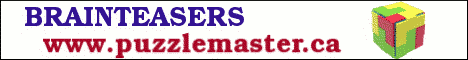# Two Equals One

## An Advanced Mathematics Lesson Starter Of The Day

 Consider two positive numbers $$a$$ and $$b$$ that are equal: $$a = b$$ Multiply both sides of this equation by $$a$$, $$a^{2}=ab$$ Subtract $$b^2$$ from both sides. $$a^{2}-b^{2}=ab-b^{2}$$ Factorise both sides $$(a-b)(a+b)=b(a-b)$$ Divide both sides by $$(a - b)$$. $$a+b=b$$ As $$a = b$$ substitute $$b$$ for $$a$$. $$b+b=b$$ Collect like terms $$2b=b$$ Divide both sides by $$b$$. $$2 = 1$$ How can this be? $$???$$

Share

Topics: Starter

How did you use this starter? Can you suggest how teachers could present or develop this resource? Do you have any comments? It is always useful to receive feedback and helps make this free resource even more useful for Maths teachers anywhere in the world.

If you don't have the time to provide feedback we'd really appreciate it if you could give this page a score! We are constantly improving and adding to these starters so it would be really helpful to know which ones are most useful. Simply click on a button below:

Excellent, I would like to see more like this
Good, achieved the results I required
Satisfactory
Didn't really capture the interest of the students
Not for me! I wouldn't use this type of activity.

This starter has scored a mean of 3.5 out of 5 based on 2 votes.

Previous Day | This starter is for | Next Day

How did you use this resource? Can you suggest how teachers could present, adapt or develop it? Do you have any comments? It is always useful to receive feedback and helps make this free resource even more useful for Maths teachers anywhere in the world. Click here to enter your comments.

Your access to the majority of the Transum resources continues to be free but you can help support the continued growth of the website by doing your Amazon shopping using the links on this page. Below is an Amazon search box and some items chosen and recommended by Transum Mathematics to get you started.For All: